Important questions of Triangle (in Geometry)

Chapter 6 Class 10 Triangles
Serial order wise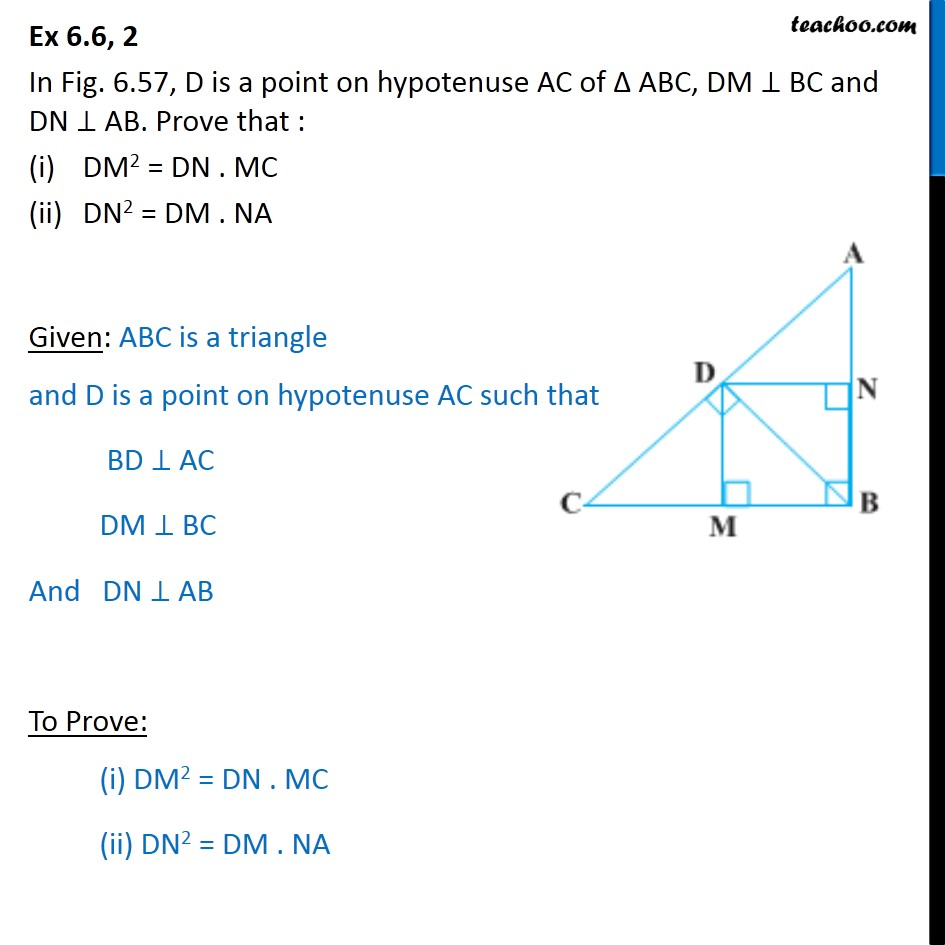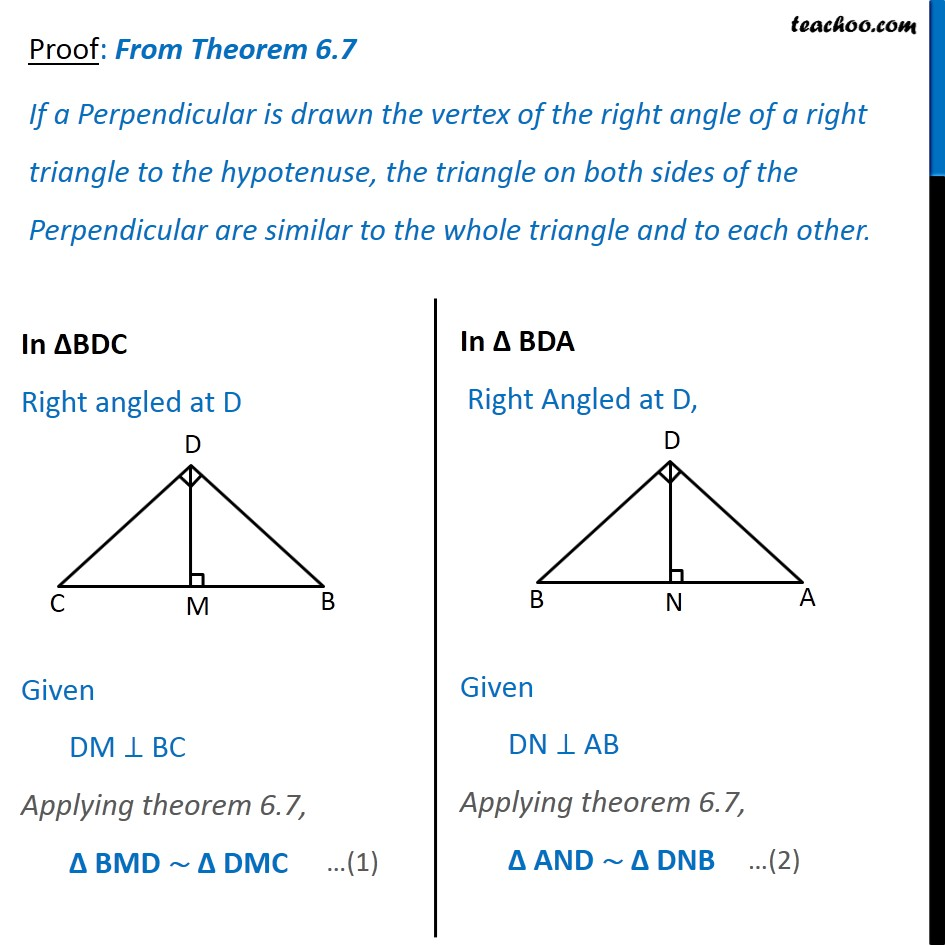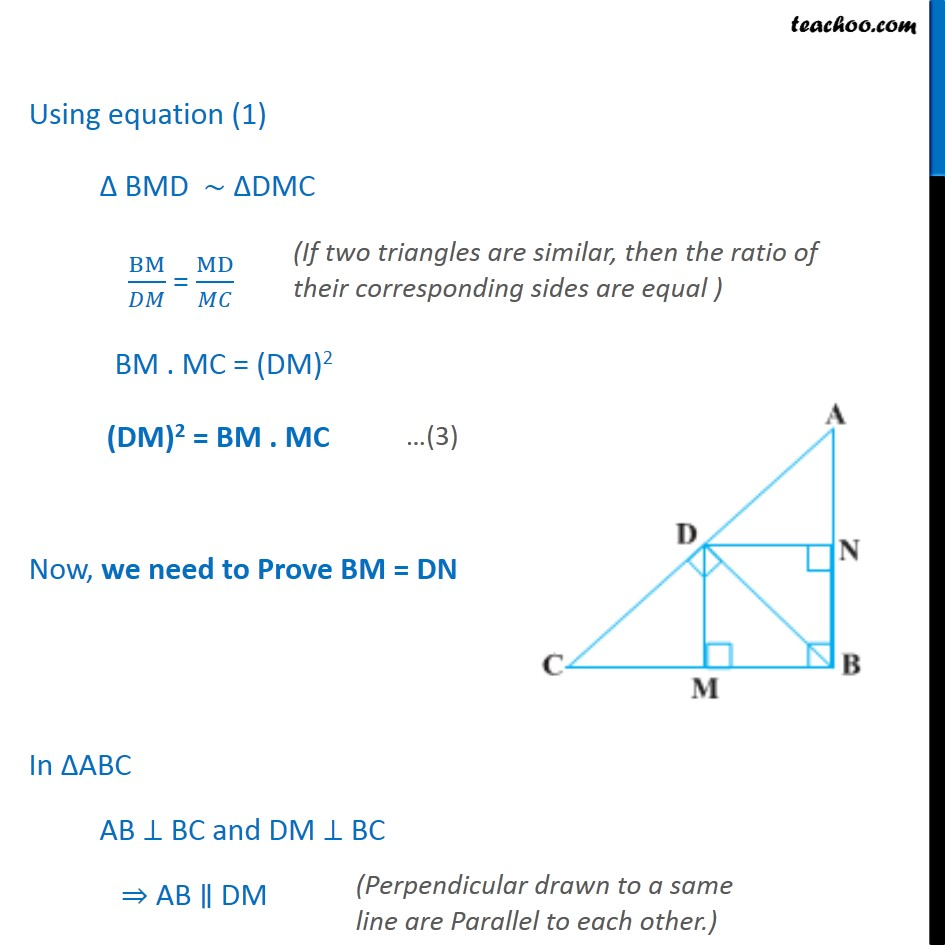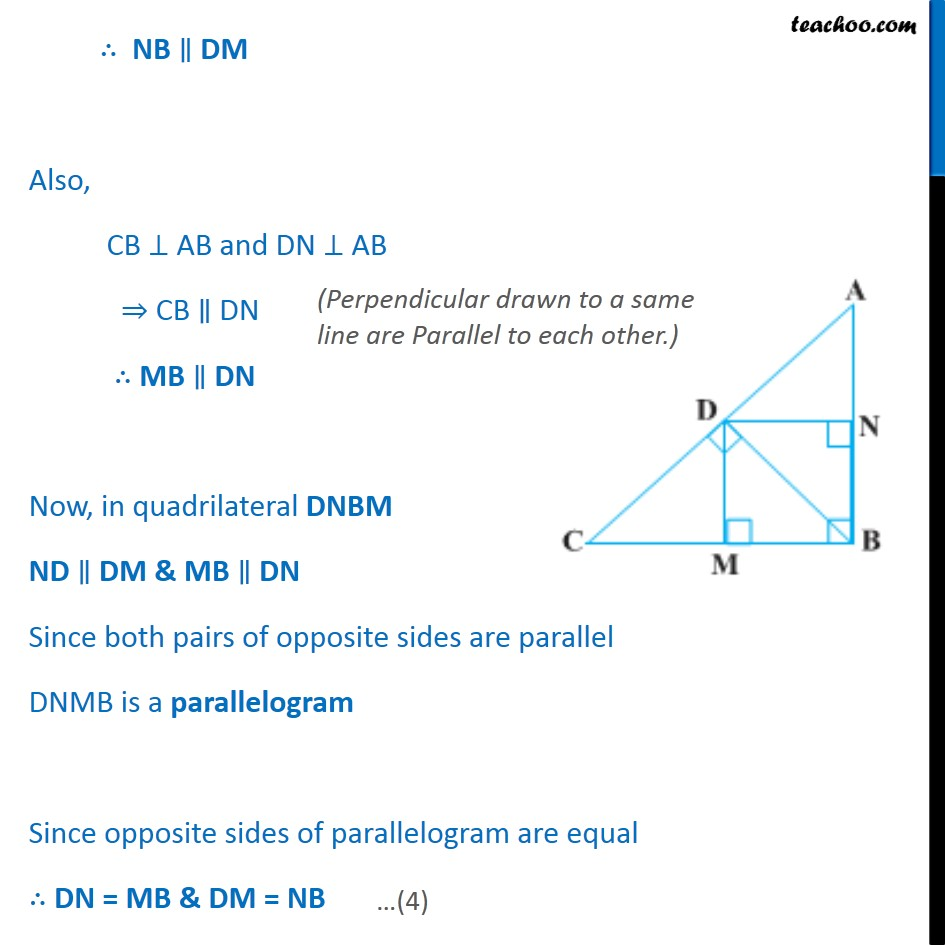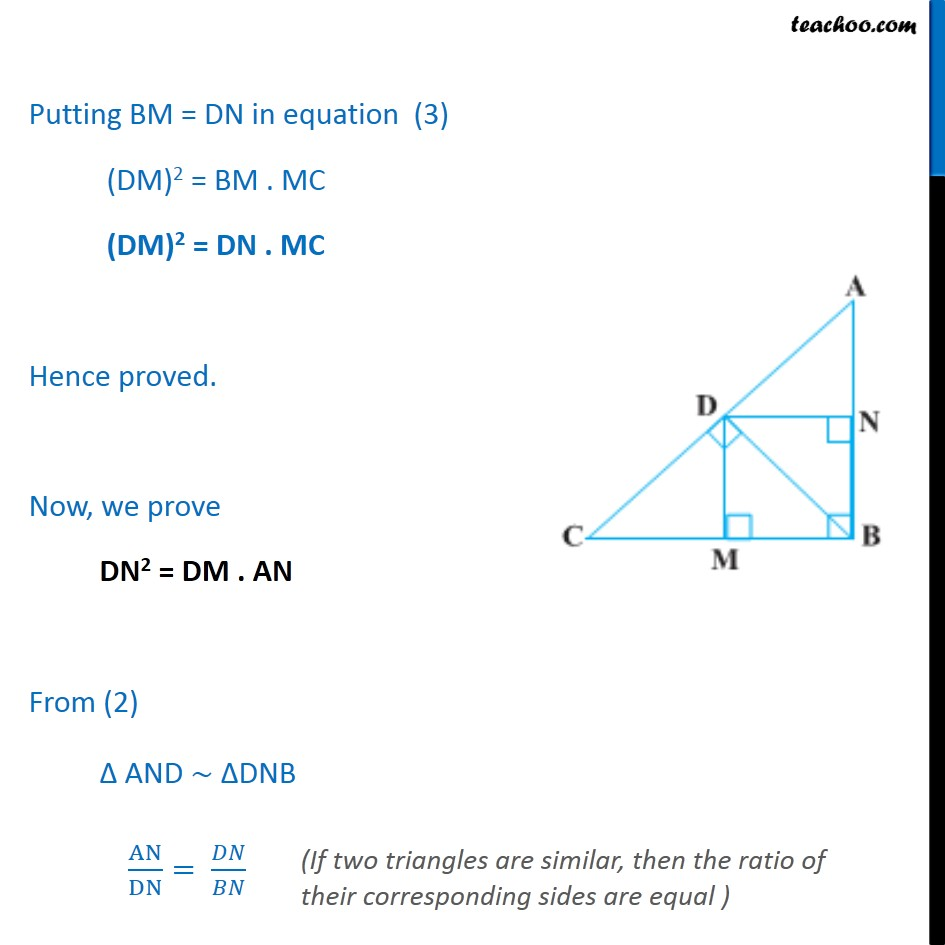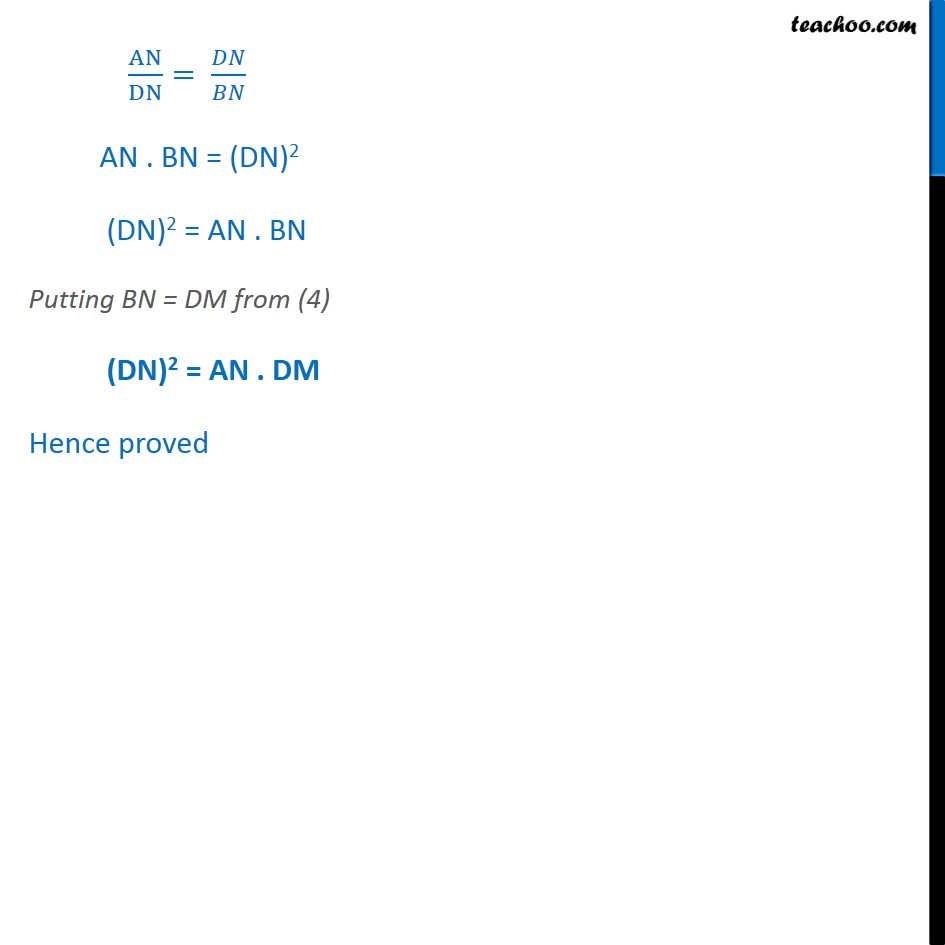Learn in your speed, with individual attention - Teachoo Maths 1-on-1 Class

### Transcript

Question 2 In Fig. 6.57, D is a point on hypotenuse AC of ABC, DM BC and DN AB. Prove that : DM2 = DN . MC DN2 = DM . NA Given: ABC is a triangle and D is a point on hypotenuse AC such that BD AC DM BC And DN AB To Prove: (i) DM2 = DN . MC (ii) DN2 = DM . NA Proof: From Theorem 6.7 If a Perpendicular is drawn the vertex of the right angle of a right triangle to the hypotenuse, the triangle on both sides of the Perpendicular are similar to the whole triangle and to each other. In BDC Right angled at D Given DM BC Applying theorem 6.7, BMD DMC In BDA Right Angled at D, Given DN AB Applying theorem 6.7, AND DNB Using equation (1) BMD DMC BM/ = MD/ BM . MC = (DM)2 (DM)2 = BM . MC Now, we need to Prove BM = DN In ABC AB BC and DM BC AB DM NB DM Also, CB AB and DN AB CB DN MB DN Now, in quadrilateral DNBM ND DM & MB DN Since both pairs of opposite sides are parallel DNMB is a parallelogram Since opposite sides of parallelogram are equal DN = MB & DM = NB Putting BM = DN in equation (3) BM . MC = (DM)2 DN. MC = (DM)2 Putting BM = DN in equation (3) (DM)2 = BM . MC (DM)2 = DN . MC Hence proved. Now, we prove DN2 = DM . AN From (2) AND DNB AN/DN= / AN/DN= / AN . BN = (DN)2 (DN)2 = AN . BN Putting BN = DM from (4) (DN)2 = AN . DM Hence proved